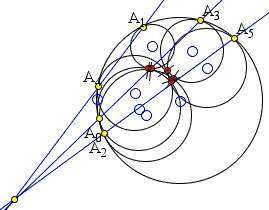# Seven Circles Theorem

The applet below illustrates the Seven Circles Theorem:

Suppose we have a chain of five circles S1, S2, . . .,S5 each touching the preceding circle in the chain, and all touching a fixed circle C. There are then two circles S6 which can be drawn touching S1, S5 and C, thus forming a closed loop of six touching circles, all touching C. Then one choice of S6 is such that the three lines joining opposite pairs of points of contact on C are concurrent.

### This applet requires Sun's Java VM 2 which your browser may perceive as a popup. Which it is not. If you want to see the applet work, visit Sun's website at https://www.java.com/en/download/index.jsp, download and install Java VM and enjoy the applet.

 What if applet does not run?

The theorem is of a recent origin and first appeared in [Evelyn, Money-Coutts, Tyrrell, pp. 31-42]. (The 68 pages book is now a bibliographical rarity and can be had for a lofty price of about \$500 - give or take a few cents.) A trigonometric proof has been published by H. Martin Cundy in 1978 and a practically elementary proof by Stanley Rabinowitz in 1975.

Solution

### References

1. H. M. Cundy, The Seven-circles Theorem, Math. Gazette. 62(1978) 200-203.
2. C. J. A. Evelyn, G. B. Money-Coutts, J. A. Tyrrell, The seven circles theorem and other new theorems , Stacey International (1974), pp. 31-42
3. S. Rabinowitz, The Seven Circles Theorem, Pi Mu Epsilon Journal, 8(1987) 441-449Rabinowitz's proof, although more restricted than the original, is a real beauty and is the one we shall present below.

The first step in the proof is a lemma found in [Coxeter] which Rabinowitz dubbed Ceva's Theorem for Chords.

### Lemma 1

Let A, B, C, D, E, and F be six consecutive points around the circumference of a circle. Then chords AD, BE, CF concur if and only if

(1)

AB · CD · EF = BC · DE · FA.

### Proof

First assume that the six points are such that the chords AD, BE, CF concur in point P. Observe the presence of three pairs of similar triangles. (In the diagram one pair is highlighted and pairs of equal angles are shown. One pair is of central angles; two pairs are inscribed subtended by the same arc.)From similar triangles, we get the proportions:

AB/DE = PA/PE
CD/FA = PC/PA
EF/BC = PF/PB
PC/PE = PB/PF.

Multiplying these together gives us the desired result.

In the other direction, suppose (1) holds but the chords do not concur. Of the three arcs ABC, CDE, EFA, at least one must be smaller than a semicircle. Let it be the arc CDE. Denote P the intersection of BE and CF. Let X be the second point of intersection of AP with the circle.By the above,

AB · CX · EF = BC · XE · FA.

Which compared to (1) gives

(2)

CX / XE = CD / DE.

If X and D are different then, say, CX > CD. But then XE < DE, so that CX / XE > CD / DE and (2) can't hold. A contradiction. Therefore, X = D and the three chords indeed concur.

The second step in the proof is the following

### Lemma 2

Let two externally tangent circles, (P) and (Q), be internally tangent to circle (C) at points A and B respectively. If the radii of circles (C), (P), and (Q) are R, p, and q, respectively, then

AB² = 4R² f(p)·f(q),

where f(x) = x / (R - x).### Proof

Triangles APM and ACD are isosceles with a common base angle at A. It follows that their other base angles (at M and D) are also equal. Therefore, CD||MP. Similarly, CE||MQ. But PMQ is a straight line and then so is DCE.

Observe also that, as inscribed angles subtended by the same arc,

∠EBA = ∠EDA and

It follows there are three pairs of similar triangles: APM/ACD, BQM/BCE, and ABM/EDM which gives the following proportions:

AB/DE = AM/EM = BM/DM.

But DE = 2R. Thus we have

 AB/2R · AB/2R = AM/EM · BM/DM = AM/EM · BM/DM = AM/DM · BM/EM = AP/CP · BQ/CQ = p(R - p) · q(R - q).

### Proof of the Seven Circles Theorem

Assume the points of tangency of circle C with circles Sk are Ak, and that rk denotes the radius of Sk, k = 1, 2, ..., 6. By Lemma 2,

(AkAk+1)² = 4R² f(rk)f(rk+1),

k = 1, 2, ..., 6, and r7 = r0. It follows that

(A1A2·A3A4·A5A0)² = 64R6 f(r0)f(r1)f(r2)f(r3)f(r4)f(r5) = (A0A1·A2A3·A4A5

which of course implies

A1A2·A3A4·A5A0 = A0A1·A2A3·A4A5

and by Lemma1 the three chords A0A3, A1A4, A2A5 are concurrent.The applet shows that the concurrency takes place in other configurations as well. For example, circles Sk may all be tangent to circle C externally. The above proof goes through in this case if the function f(x) is redefined: f(x) = x/(R + x).In a special case where circles S1, S3, S5 expand into straight lines, the configuration becomes that of the incircle of a triangle with three additional circles inscribed into curvilinear triangles cut off by the incircle. If the radii of the latter shrinks to 0, the remaining points of tangency are none other than the intouch points and the point of concurrency is the point of Gergonne.

As can be grasped from the diagrams below, the theorem holds in a more general setting where the points of tangency Ak do not form a convex hexagon.### References

1. H. S. M. Coxeter, Problem 527, The Mathematics Student 28 (1980) 3.